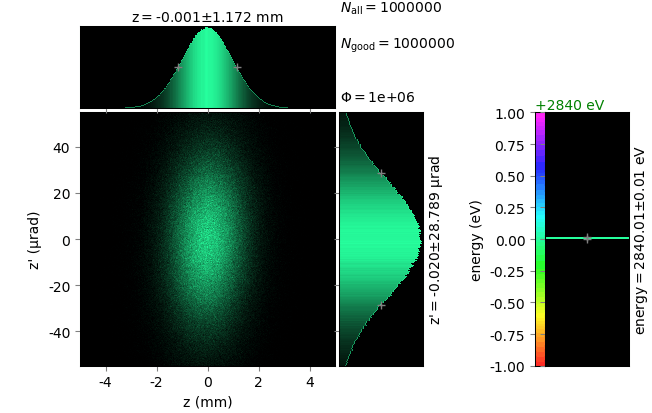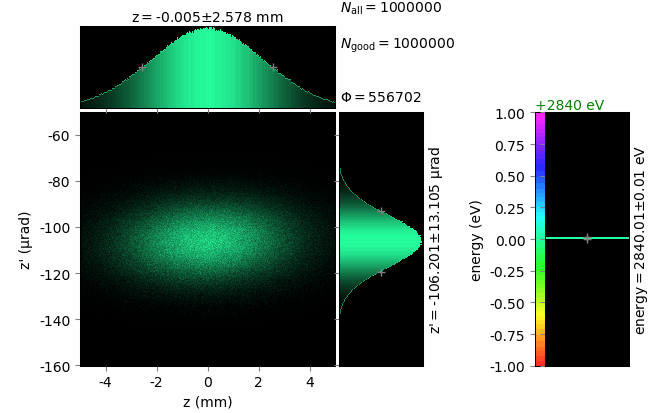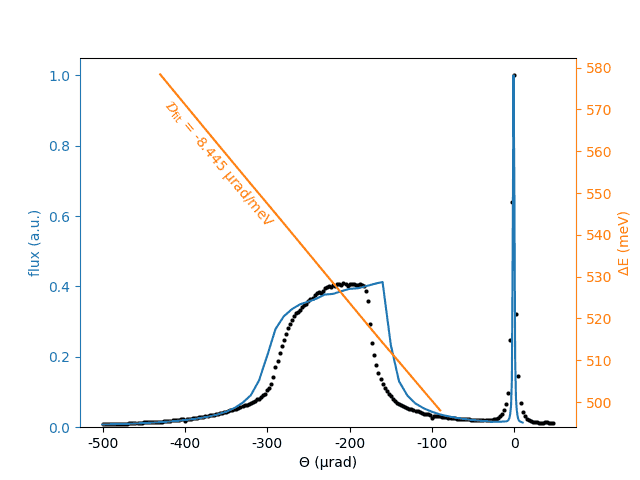# Tests for Optical elements¶

## Reflection from asymmetrically cut crystal¶

A monochromatic beam of E = 2840 eV is diffracted by a Si111 crystal with θB= 44.132 deg and α = -20.054 deg, which results in b = -0.453.

The phase space volume in the beam before and after the crystal is indeed invariant, whereas the linear and angular sizes have scaled with the ratio equal to b or 1/b. The product FWHM(z)×FWHM(z’) = 135 nmrad in both cases. Notice also the deviation of the diffracted beam direction from the exact departure angle 2θB seen on the exit screen which is put normally to 2θB (i.e. z’=0 at the exact departure at 2θB). The ray-traced deviation -106 µrad is close to the calculated refractive shift -105 µrad.## Reflectivity from highly asymmetric crystal in backscattering¶

A test for the optical scheme from [ShvydkoPRL2006], Fig 3.The blue curve was ray-traced by xrt. The dotted curve is a reconstruction from Fig. 4 of [ShvydkoPRL2006]. The dispersion rate was calculated from a linear fit to the energy-angle line (orange) and is similar to that reported in [ShvydkoPRL2006] as 8.5 µrad/meV.

 [ShvydkoPRL2006] (1, 2, 3) Yu. V. Shvyd’ko, M. Lerche, U. Kuetgens, H. D. Rüter, A. Alatas, and J. Zhao, Phys. Rev. Lett. 97 (2006) 235502 (DOI: 10.1103/PhysRevLett.97.235502).